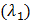# The focal length of an equi-convex lens is greater than the radius of curvature of any of the surfaces. Then the refractive index of the material of the lens is a) Greater than zero but less than 1.5 b) Greater than 1.5 but less than 2.0 c) Greater than 2.0 but less than 2.5 d) Greater than 2.5 but less than 2.0

## Question ID - 152813 :- The focal length of an equi-convex lens is greater than the radius of curvature of any of the surfaces. Then the refractive index of the material of the lens is a) Greater than zero but less than 1.5 b) Greater than 1.5 but less than 2.0 c) Greater than 2.0 but less than 2.5 d) Greater than 2.5 but less than 2.0

3537

 (a) Focal length of lensFor equi-convex lens,Focal length of convex lens is positive. So,cannot be negative, hence should be greater than zero but less than 1.5
 With respect to air critical angle in a medium for light of red colouris. Other facts remaining same, critical angle for light of yellow colourwill be a)b) More thanc) Less thand)